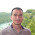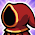## Friday, June 01, 2018

### BQP not in the Polynomial-Time Hierarchy in Relativized Worlds

The quantum complexity world is a rocking with the paper released yesterday by Ran Raz and Avishay Tal, Oracle Separation of BQP and PH, resolving a question open since quantum complexity got its start over two decades ago. Scott Aaronson's 2010 paper that sets some of the groundwork for the Raz-Tal result gives a nice overview of the question.

All of you readers should be familiar with the P v NP question. P is the set of problems efficiently solvable by a deterministic computer. NP are the problems for which there exists a witness that we can verify quickly. The polynomial-time hierarchy (PH) is the constant alternation generalization of NP that has played a major role in computational complexity So why don't we talk about the P vs PH question? That's just equivalent to P vs NP.

BQP is the the class of problem efficiently solved by a quantum computer. Since P sits in BQP which sits in PSPACE we can't prove outright any separations for BQP without separating P from PSPACE. We can though get an understanding of complexity by allowing the machines access to the same oracle and seeing what we can separate. We already knew BQP doesn't sit in NP relative to an oracle. Same was true for BPP (efficient probabilistic computation) but BPP is in PH for all oracles as are constant round interactive proofs. So we might expect we can have BQP in PH, BQP solvable with constant alternations, relative to all oracles. Raz and Tal refute that hypothesis.

Raz and Tal's proof builds on the Forrelation concept developed by Aaronson (under the name Fourier Checking) and extended by Aaronson and Ambainis. The oracle isn't complicated: Pick n numbers x1,...,xn each xi chosen from a normal distribution with mean 0 and variance a small ε > 0. Let y=y1,...,yn be the Hadamard transform applied to x1,...,xn. You then probabilistically round the x's and y's to 1 or -1. A quantum machine can distinguish this distribution from uniform using the fact that quantum machines can do Hadamards easily and Raz and Tal use Fourier show that low-depth alternating circuits (that capture PH) can't distinguish so easily. The paper is well-written if you want details.

A few questions for further directions:
• Can you show a relativized world where P = NP (=PH) but P ≠ BQP? I'd suspect it will be a messy but not too hard generalization of this paper.
• How far can you push the Raz-Tal result, i.e., for what function f(n) can we show that BQP cannot be solved by f(n)-alternating polynomial-time Turing machines. Can you show f(n) = nΩ(1)?
Also see Scott's personal take on this new result.

1.Hi Lance,

Thank you for your wonderful post. You ask how many alternation can we handle? The answer is that using Theorem 7.4, our lower bound would still hold for any f(n) = o(n/log(n)) alternations.

Best, Avishay.

2.wasn't this problem featured in a recent episode of rick and morty?

3.What do you think about BH